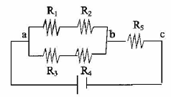Problem: A combination of resistors, shown in the accompanying figure, is attached to a 10 V battery. Assume R1 = R2 = 5 Ω, R3 = R4 = 15 Ωand R5 = 20 Ω.a) Find the equivalent resistor R1.b) Find the current through the resistor  R 3.c) Find the current through the resistor R 2.d) Find the voltage drop across the resistor R 2.e) Find the voltage drop across the resistor R 5.

FREE Expert Solution
80% (312 ratings)
Problem Details

A combination of resistors, shown in the accompanying figure, is attached to a 10 V battery. Assume R1 = R2 = 5 Ω, R3 = R4 = 15 Ωand R5 = 20 Ω.

a) Find the equivalent resistor R1.

b) Find the current through the resistor  R 3.

c) Find the current through the resistor R 2.

d) Find the voltage drop across the resistor R 2.

e) Find the voltage drop across the resistor R 5.